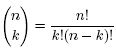Problems

# Binomial coefficients

Time limit 1 second
Memory limit 128 MiB

Gunnar is quite an old and forgetful researcher. Right now he is writing a paper on security in social networks and it actually involves some combinatorics. He wrote a program for calculating binomial coefficients to help him check some of his calculations.

A binomial coefficient Ck_n is a numberwhere n and k are non-negative integers.

Gunnar used his program to calculate Ck_n and got a number m as a result. Unfortunately, since he is forgetful, he forgot the numbers n and k he used as input. These two numbers were a result of a long calculation and they are written on one of many papers lying on his desk. Instead of trying to search for the papers, he tried to reconstruct the numbers n and k from the output he got. Can you help him and find all possible candidates?

## Input data

First line contains the number of test cases, at most 100. Each test case is one line with an integer m (2m10^15) - the output of Gunnar's program.

## Output data

For each test case print two lines. The first line must contain the number of ways of expressing m as a binomial coefficient. Print in the second one line all pairs (n, k) that satisfy Ck_n = m. Order them in increasing order of n and, in case of a tie, order them in increasing order of k. Format them as in the sample output.

## Examples

Input example #1
2
2
15

Output example #1
1
(2,1)
4
(6,2) (6,4) (15,1) (15,14)

Source 2011 ACM Northwestern Europe (NWERC), Problem A